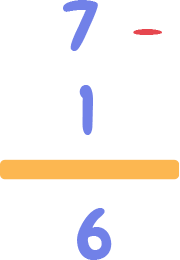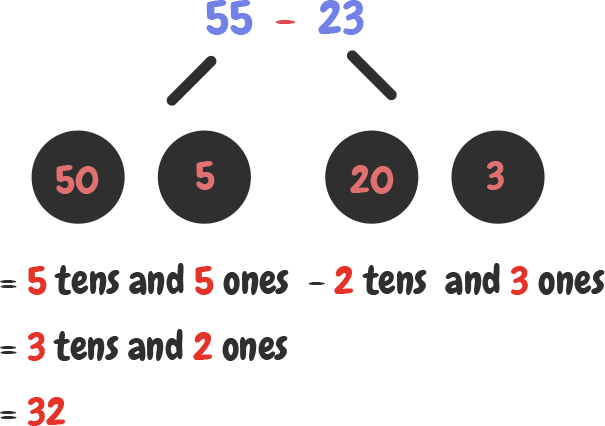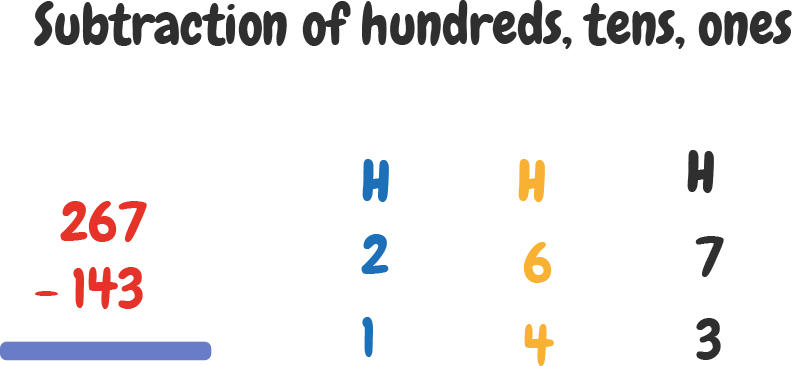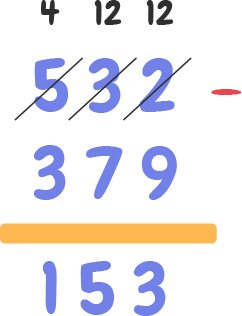Courses
Courses for Kids
Free study material
Free LIVE classes
More

# Easy Subtraction Sums with Workbook and Stories## Class 2 Subtraction Sums Workbook with Answers: Story of Riya and her Parrot

Do you know what subtraction is? What if I give you 10 apples and your brother took 2 apples from you. How many apples will you be left with? Well, you will be left with 8 apples. How did I know that? Voila! Here is the magic. Math provides us with a lot of interesting concepts and one of them is called “subtraction”. Do you know what this is all about? Let’s read Riya’s story and understand how it works.

### Riya lives with her parrot friend “Kuku” and one day she offered her 7 green chilies out of which the parrot ate only 1. So, how many chilies are left with the parrot?Here, we will determine the number of chilies left by using a very interesting concept.

Please remember that when we are asked “How many are left or How many remain?” is where we need to perform the subtraction operation. So, now we will explain Subtraction for Class 2?

Now, let us understand how many chilies are remaining for the above story.So, we get the answer to our question as 6. Therefore, 6 chilies were left after the parrot ate only 1.

Now, let us see another story on subtraction sums for Class 2 to understand subtraction better. Moving forward, we will also understand how subtraction is actually performed. Now, we need to see how the subtraction is done for one-digit numbers, for a two-digit number and a one-digit number, for 2 two-digit numbers, and so on.

### How do we perform subtraction on numbers?

One day, my best friend Sonali was invited to an award ceremony. 55 guests were invited to the ceremony, out of which 23 turned up. How many guests could not turn up at the ceremony? Well, to find the number of guests who could not turn up at the ceremony, we need to understand one of the very interesting concepts called “place value”.

Place Value Concept

Here, the sequence of placing each digit in numbers 55 and 23 is as follows:

 Tens Ones 5 5

 Tens Ones 2 3

Here, Ones place means 5 * 1, and 5 at tens place means 5 * 10 = 50

So, 50 + 5, we get 55.

Simialty, 3 at Ones place means 3 * 1 = 3 and 2 at tens place means 2 * 10 = 20, we get 20 + 3 = 23.

Now, let us learn how the subtraction is performed for the guests who didn’t turn up at the ceremony.

 Guests Invited Attended the ceremony Didn’t Attend  (subtraction) 55 23 55 - 23 = 32

After we have got the result, we write as per the place value, which is as follows:

 Tens Ones Deciding the place value Numer obtained 3 2 3 * 10 + 2 * 1 32

Below is the visual presentation of this table.### Subtraction of Three-Digit Numbers

Yesterday, my friends went to a very big library, and you know what, they came to know that 267 people visit this library every day, however, on average, only 143 people have a membership with this library.

My friends told me this story, and I was very shocked to hear such a big number, but we wanted to find how many people didn’t have a membership. Well, for this, we will have to again use the “Place Value Concept”.

Placing the value 267:

 Hundreds Tens Ones 2 6 7

2 hundreds = 2 *  100  =200

6 tens = 6 * 10 = 6

7 ones =7 * 1 = 7

Similarly, for 143:

 Hundreds Tens Ones 1 4 3

1 hundred = 1 *  100  = 100

4 tens = 4 * 10 = 40

3 ones = 3 * 1 = 3

Now, let us find the number of non-paid membership people by subtraction.Hundreds (H) Tens (T) Ones (O) Subtraction 2 6 7 H = 2 - 1 = 1T = 6 - 4 = 2O = 7 - 3 = 4 1 4 3

So, subtract hundreds from hundreds, tens from tens, and so on. We can see that 124 persons were non-paid members of the library.

Isn’t it so simple? Yes, it is. But what if we have to arrange a few more players for our cricket team? Let’s take a look. Assume that in an 11-member team, seven of your teammates couldn’t play cricket, so how many more members would you need to make it to 11? Here, we will understand subtraction with borrowing for class 2.

### Subtraction with Borrowing for Class 2

In the above example of 11 and 7, we have the following arrangement:

 Tens Ones 1 1 0 7

Now that we subtract 7 ones from 1 ones, we notice that 1 < 7, so what we do is take another 1 from 1 tens and make a single digit in the Ones Place to a two-digit.

 Tens Ones Borrow 1 from Tens 1 1 0 11 0 7 0 7

Now, 11-7 gives us 4. So, 4 teammates are required to complete the cricket team.

A similar example for subtraction sums for class 2 with borrowing for three-digit numbers is as follows.### Story 2 on the Subtraction of Numbers with Borrowing

Some students were asked to participate in a drawing competition and they had to participate in a team. Here, if you look at the image below, some kids agreed to participate in a team.

Similarly, there were some more teams that participated in the same competition.

Now, we have to see what difference do we find in the number of participants in both the teams, i.e., Team 1 and Team 2. This we can do by performing subtraction between the number of participants shown below.

 Team 1 Team 2 Difference in Participants 11 5 6 - 4 = 2

Now, we see that 11 =  1 tens and 1 ones

5 =  0 tens and 5 ones

So, let us borrow 1 from 11 and we get another form:

 Tens: Team 1 Ones: Team 2 Difference in participants 0 11 11 - 6 = 5 0 5

You can see that 11 participants were there in team 1 and 5 in team 2. So, the difference in the members was 6.

So, this was all about Class 2 Subtraction. We started with subtraction of two, and three-digit numbers and proceeded with the concept of borrowing.

Last updated date: 24th Sep 2023
Total views: 162.6k
Views today: 1.62k

## FAQs on Easy Subtraction Sums with Workbook and Stories

1. Are addition and subtraction the same?

No. One day, you wanted to watch the first show of the “ABC” movie and the person at the ticket counter said that you can purchase only “3” tickets out of 5, as the rest of the tickets are reserved. So, here, the other two tickets can be determined by subtraction, not addition.

In the case of the addition of two numbers, it would have like 3 tickets free with 5 tickets, you get 8 tickets, which is not the scenario here.

Thus, addition and subtraction are two different things.

2. How do you teach subtraction of tens, hundreds, and thousands?

33 tens = 3 tens + 3 ones and 21 =  2 tens + 1 ones

So, 3 tens - 2 tens = 1 tens, and  3 ones - 1 ones = 2 ones

We get our answers as 1 tens 2 ones, i.e., 12.